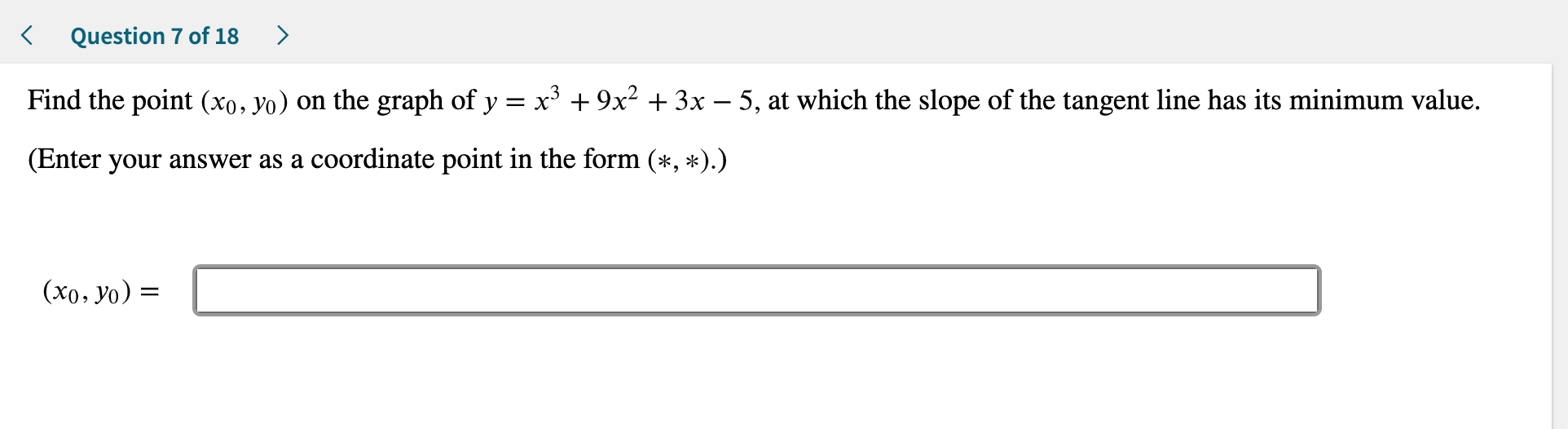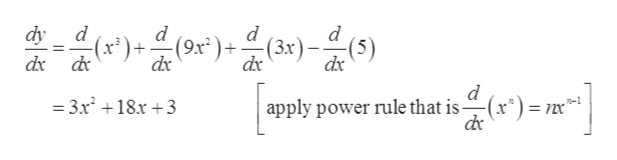# Question 7 of 18Find the point (xo, yo) on the graph of yx39x2 3x - 5, at which the slope of the tangent line has its minimum value(Enter your answer as a coordinate point in the form (*, *).)(xo, yo)=

Question
39 viewshelp_outlineImage TranscriptioncloseQuestion 7 of 18 Find the point (xo, yo) on the graph of y x39x2 3x - 5, at which the slope of the tangent line has its minimum value (Enter your answer as a coordinate point in the form (*, *).) (xo, yo)= fullscreen
check_circle

Step 1

Given:

Step 2

To find the point (x0,y0) on the graph where slope of tangent has its minimum point , first calculate the slope of tangent line.

Slope of tangent line is given by the first derivative of the function.help_outlineImage Transcriptionclose음미음여가음메음이 )()(3x)) d (9x* )+ dx d (5) dy d d dx d d apply power rule that is (x*) =, 3x 18x +3 -1 fullscreen
Step 3

Let tangent of line define...

### Want to see the full answer?

See Solution

#### Want to see this answer and more?

Solutions are written by subject experts who are available 24/7. Questions are typically answered within 1 hour.*

See Solution
*Response times may vary by subject and question.
Tagged in

### Calculus